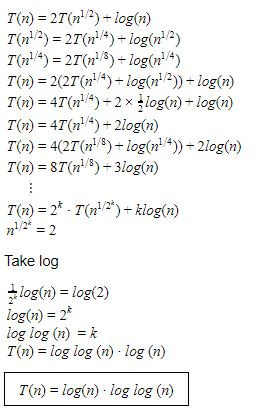## Recurrence-Relations

 Question 1

The solution of recurrence relation : T(n) = 2T(sqrt(n)) + lg(n) is

 A O(lg (n) lg(n)) B O(lg (n)) C O(n lg (n)) D O(lg (n) lg(lg(n)))
Algorithms       Recurrence-Relations       UGC-NET DEC Paper-2
Question 1 Explanation:There is 1 question to complete.
PHP Code Snippets Powered By : XYZScripts.com